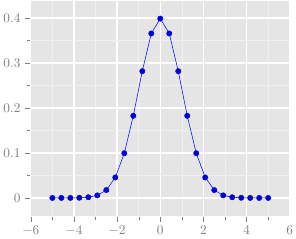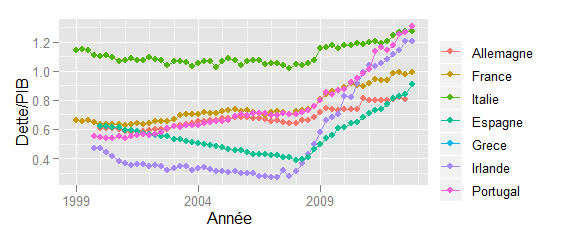# Plotting like ggplot2 with TikZ/Latex

I appreciate the graphics drawn by the ggplot2 package with R, results are nice and elegant. I decided to imitate the style of ggplot2 with TikZ. Here is a working example:

% Gauthier Vermandel 2014 \documentclass{standalone} \usepackage{pgfplotstable}     \definecolor{mygrey}{RGB}{229,229,229} \definecolor{mygrey2}{RGB}{127,127,127} \definecolor{mygrey3}{RGB}{240,240,240}     \pgfplotsset{ axis background/.style={fill=mygrey}, tick style=mygrey2, tick label style=mygrey2, grid=both, xtick pos=left, ytick pos=left, tick style={ major grid style={style=white,line width=1pt},minor grid style=mygrey3, tick align=outside, }, minor tick num=1, }     \begin{document} \begin{tikzpicture}   \begin{axis}[draw=white]   \addplot {1/sqrt(2*pi)*exp(-x^2/2)};   \end{axis}   \end{tikzpicture} \end{document}# Converting Scientific Workplace to Matlab by copy/pasting

For my PhD I’d been needing  a Scientific Workplace to Matlab/R converter to test fastly different steady states. I wrote that converter to get in one clic some matlab code directly from scientific workplace. In the picture, i give an example with Hansen’s steady states: you just have to copy from your scientific workplace document and paste it in the LaTeX2Matlab windows. I wrote the converter in PHP directly.
→direct link to LaTeX2Matlab

# R Codes from my post about the Reinhart-Rogoff working paper

[latexpage]In this post, I explain how to make two graphs from my post dealing with the article of Reinhart and Rogoff (french). The first figure is simple plot while the other is non parametric estimate by Splines.